Published: 15 March 2019

# Analysis of diesel engine isolation system with piston slap

Song Yuchao1
Liao Jianbin2
Yu Hongliang3
1, 2, 3Marine Engineering College, Dalian Maritime University, Dalian, China
2, 3Marine Engineering College, Jimei University, Xiamen, China
Corresponding Author:
Song Yuchao
Views 233

#### Abstract

An isolation system of diesel engine with cylinder gap was modeled to deeply study the effect of piston shock on the whole vibration system. With the diesel engine rotates at rated speed, the center of engine body mass moves in plane with an obvious ellipse, and the magnitude is enlarged high to 3.5 times of the origin. All the bottom three directional springs have the corresponding response with the fundamental frequency signal, and a characteristic frequency band, as the piston slap is considered.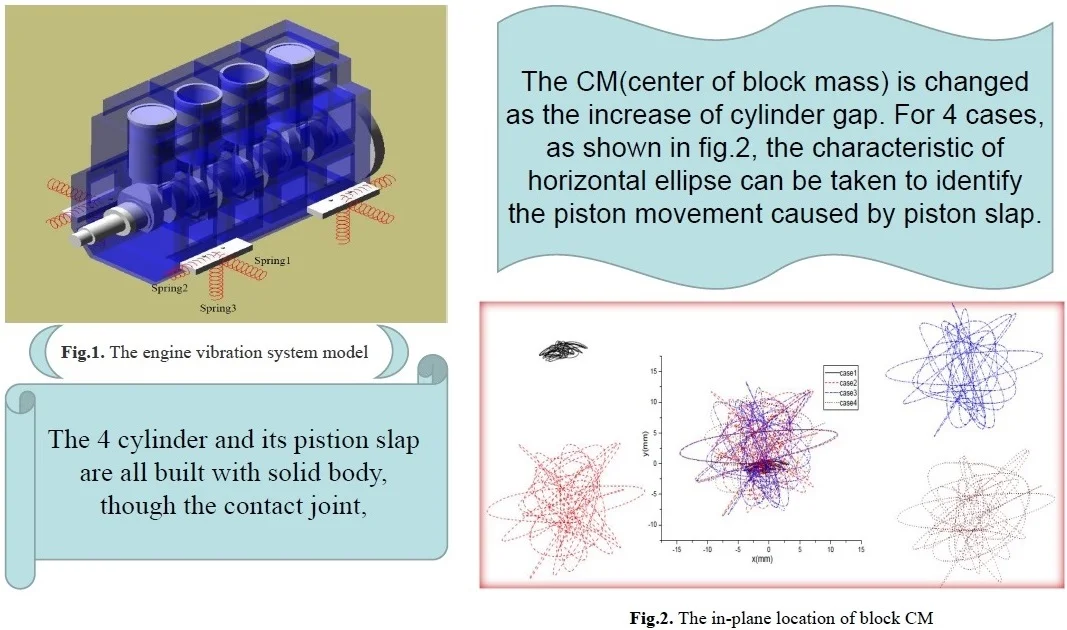#### Highlights

• A multi-cylinder model was built, considering the piston slap
• The isolation and vibration response can simultaneously be calculated, with the variation of control parameters
• The resluts can be used to identify the piston slap in rotation

## 1. Introduction

Diesel engine produces a certain amount of noise in operation. The main noise is mechanical noise generated by combustion process. Piston is the main moving part of diesel engine which produces mechanical noise. It bears gas force and inertia force when it works. In diesel engine, because of the gap between cylinder liner and piston, piston moves reciprocating along the axis of cylinder liner, it has weak translation and rotation inside the cylinder liner at the same time, which is the second-order motion of piston. When the speed is high or the pressure of the cylinder is high, it can produce considerable scratching force and knocking force, which results in scratching or knocking on the cylinder liner. These two forces are a very important source of excitation for generating the mechanical noise of the diesel engine . Therefore, it is very important to study and analyze the motion of piston for designing low-noise diesel engine.

For a long time, the research on vibration characteristics of diesel engine has been done by many works, including the piston slap and its noise identification [2, 3]. Nowadays, with the developing of computer-aided engineering, the numerical computation can be easily performed, such as the whole engine isolation vibration system model and its analysis . In abroad, not only the piston slap, but also its effect on the cavitation of wet cylinder liner are the main research contents [5-10].

In this paper, for deeply study the piston slap and later analysis of its effect on the cavitation in diesel engine , a diesel engine isolation system with cylinder gap is modeled in ADAMS. The specific research and main content are to build the movement of the diesel engine parts and establish the contact constraint of piston and cylinder liner, and then analyze the effect of piston impact on the system response.

## 2. Formula analysis of piston shock

For a piston moving in the cylinder, the side trust ${F}_{st}$ is always composed of three parts, including the component force of gas force ${F}_{g}$, reciprocating inertia force ${F}_{OG}$ and gravity force ${F}_{G}$ of reciprocating parts, which is expressed as:

1
${F}_{st}=\left({F}_{g}+{F}_{OG}+{F}_{G}\right)\mathrm{*}\mathrm{t}\mathrm{g}\beta ,$

where, the $\beta$ is the angle between connecting rod and cylinder center line. But it is not exact, when considering the gap $\mathrm{\Delta }$ between the piston and cylinder liner.

As shown in Fig. 1, the piston with center mass of $O\text{'}$, has a rock around the center of pinhole $O$, the swing angle is $\theta$, it has:

2
$\theta \cong ac\mathrm{s}\mathrm{i}\mathrm{n}\left(\mathrm{\Delta }/l\right),$

where, the $l$ is the distance of center $O$ to the piston top surface.

Given the piston is a rigid body, the impact of piston in cylinder is not a usual direct cylindrical surface shock, there is a impact contact process from point to line and surface. A simple analytical formula like Eq. (1) is not able to give the accurate description.

For the slap vibration system of piston, the multibody dynamic modal can be expressed as:

3
$M\left(q,t\right)\stackrel{¨}{q}+{\mathrm{\Phi }}_{q}^{T}\left(q,t\right)\lambda -Q\left(q,\stackrel{˙}{q},t\right)=0,$
4
$\mathrm{\Phi }\left(q,t\right)=0,$

where, $\mathrm{\Phi }$ is the constraint equation of coordinate matrix $q$, ${\mathrm{\Phi }}_{q}$ is the Jacobian matrix and $\lambda$ is the lagrange multiplier, $q$, $\stackrel{˙}{q}$, $\stackrel{¨}{q}\in {R}^{n}$ are the position, velocity and acceleration vectors of the system, $t$ is the time step, $M$ is the inertia matrix of mechanical system, $Q$ is the excited load vector. All the above equations can be solved by different integral technique in ADAMS.

Fig. 1The piston movement in cylinder with gap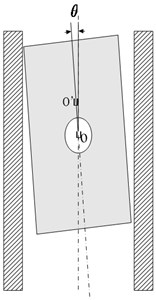Fig. 2The engine vibration system in ADAMS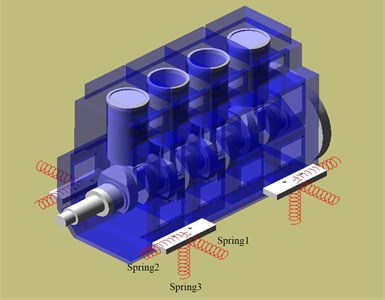## 3. Modelling of diesel engine isolation system

The main diesel engine parts are modeled in Pro/E, including the piston, connecting rod, engine body, crankshaft and so on. Each IGES file is imported into ADAMS. The whole body of diesel engine are built by the constraint pairs according to the working cases. In this paper, the four contact pair case of piston and cylinder are studied, which are shown in Table 1. The isolators are modeled by spring-dampers, their parameters can be settled as the constants to model the linear case, as the vertical spring $k=$ 1×106N/m to model the elastic support, other two springs have the same parameters $k=$ 1×1010N/m to model the relative rigid support, and all the spring has the damp $c=$ 0. And the constant rotating speed 1000 rpm is added to the rotating shaft.

The vibration system sketch is shown in Fig. 2, there are three spring isolators located at the four bottom supported points. The deformation and vibration phenomenon of spring 1, spring 2 and spring 3 are the analyzed. The spring 1 is taken to sense the horizontal vibration, the spring 2 to sense the longitudinal vibration and the spring 3 to sense the vertical vibration.

Table 1The impact analysis case

 Case 1 Case 2 Case 3 Case 4 Cylinder gap 0 0.01 mm 0.02 mm 0.03 mm

## 4. Analysis of system vibration

During 0-1 s, the vibration system is calculated with step size of 0.0004 s. As the good convergence, the results can also be exported out to do further study.

### 4.1. the engine body vibration response

The CM (center of block mass) is changed as the increase of cylinder gap. The in-plane location curves are shown in Fig. 3, with a different range. The swing range with horizontal ellipse has a magnitude 7.26 mm, 25.62 mm, 25.57 mm, and 25.68 mm for the case 1, case 2, case 3 and case 4, respectively. It is obviously that the swing range is enlarged with 3.5 times, and the case 3 with 0.02 mm gap has the relatively small value, with a decrease of 0.20 % and 0.43 compared to the case1 of 0.01 mm gap and case4 of 0.03 mm gap. The characteristic of horizontal ellipse can be taken to identify the piston movement caused by slap.

Fig. 3The in-plane location of block CM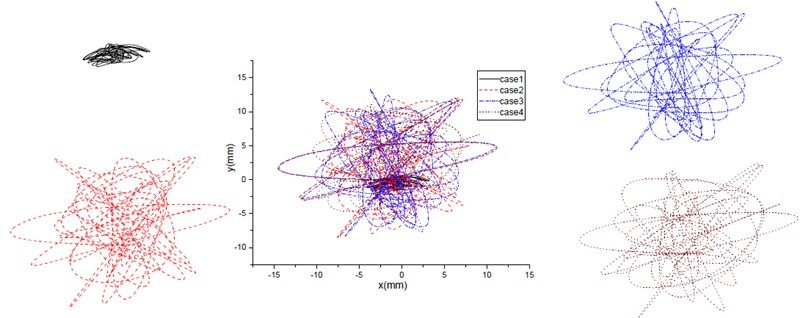All the time deformation responses of three springs are shown in Fig. 4, Fig. 5 and Fig. 6. From the amplitude of such spring, it is known that the deformation of spring is amplified by the impact of piston. The deformation of spring in case 1 with no cylinder gap, is like a sinusoidal wave. As the case with cylinder gap, the deformation of spring is like harmonic wave.

Fig. 4The deformation of horizontal spring 1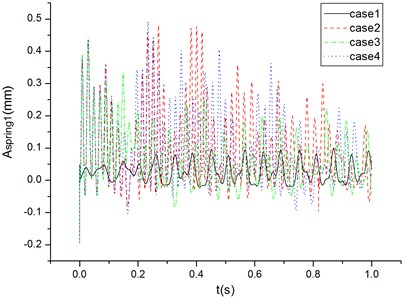Fig. 5The deformation of longitudinal spring 2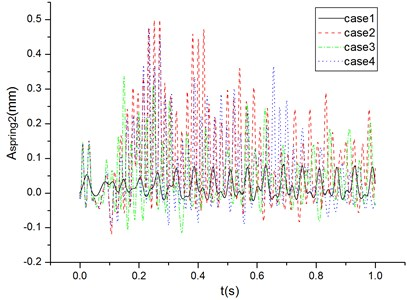After the FFT of spring deformation, the frequency spectrum of each spring are shown in Fig. 7, Fig. 8, and Fig. 9 respectively. In Fig. 7, the horizontal spring1 with no cylinder gap, has three main frequencies, as 9.77 Hz, 16.48 Hz, and 32.96 Hz. In Fig. 8, the longitudinal spring 2 with no cylinder gap has two main frequencies, as 16.48 Hz and 32.96 Hz. In Fig. 9, the vertical spring 3 with on cylinder gap, has only one main frequency of 16.48 Hz. As the rotating speed 1000 rpm, the fundamental excitation frequency is about 16.67 Hz. So, the fundamental frequency can be easily sensed by the spring vibration signal.

When there is a gap between cylinder and piston, the spectrum change a lot, with a high magnitude and a series of frequency bands. As for the horizontal spring 1, when the cylinder gap is 0.01 mm, the main frequency is a low value of 6.82 Hz, and a high frequency band from 37.22 Hz to 59.55 Hz. When the cylinder gap is 0.02 mm, there are several main frequency bands, including 2.49 Hz, 6.20 to 6.82 Hz, 28.68 Hz to 39.28 Hz, and 49.88 Hz. As for the case 4 with cylinder gap of 0.03 mm, it is similar to the case 2. So, it is known that all the spectrum of horizontal spring1 have an obvious high frequency band after the cylinder with gap, with the maximum magnitude at 52.73 Hz, 34.92 Hz, and 44.54 Hz for the case 2, case 3 and case 4 respectively. The frequency increase ratio is 219.96 %, 111.89 %, and 168.02 % respectively compared to the sensed main frequency value of 16.48 Hz.

Fig. 6The deformation of vertical spring 3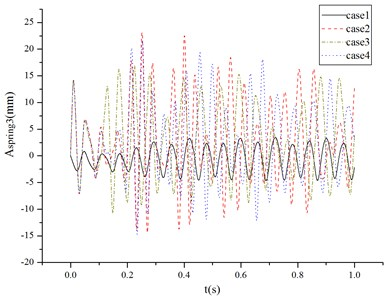Fig. 7The frequency spectrum of spring 1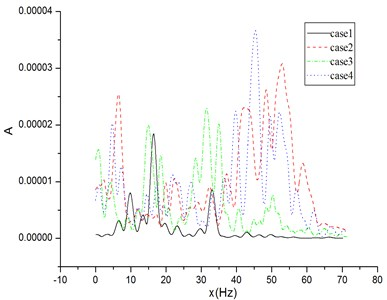Fig. 8The frequency spectrum of spring 2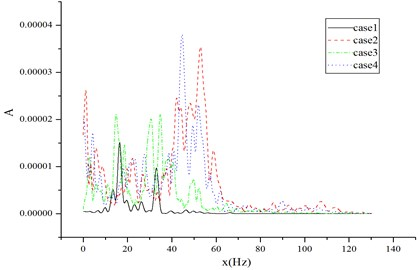Fig. 9The frequency spectrum of spring 3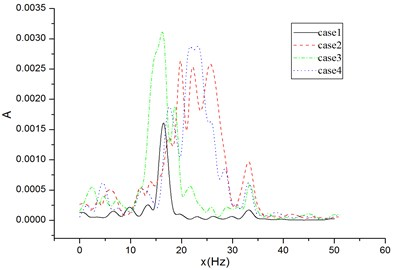The longitudinal spring 2 also has the like trend with spring1. As for the vertical spring 3, there are no low frequency band emerging with the cylinder gap, the magnitude among the fundamental frequency 16.48 Hz is surged with about 2 times. The main frequency of case2 with cylinder gap 0.01 mm is 19.85 Hz, 22.33 Hz, and 25.43 Hz. It is 16.21 Hz, and 21.65 Hz to 23.51 Hz for the case 3 with cylinder gap of 0.02 mm and case4 with cylinder gap of 0.03 mm.

## 5. Conclusions

This paper provides a model of diesel engine isolation system with the cylinder gap. The multi-body dynamic model with spring isolators is analyzed through ADAMS. There is an ellipse profile during each case with cylinder gap, caused by the impact between the piston and cylinder. Given the rotating condition, the position of engine body mass center has a larger circle in plane, due to the increase of cylinder gap. The bottom elastic supports of spring, have the corresponding characteristic response. That frequency spectrum characteristics can be used to easily sense the cylinder gap.

#### Acknowledgements

The paper is supported by the Fundamental Research Funds for the Central Universities and is also under the assistance of Fujian Province Key Laboratory of Ship and Ocean Engineering, and Fujian Engineering Research Center of Marine Detecting and Remanufacturing.